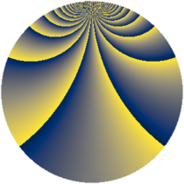# Properties

 Label 1339.2.bpLevel $1339$ Weight $2$ Character orbit 1339.bp Rep. character $\chi_{1339}(25,\cdot)$ Character field $\Q(\zeta_{102})$ Dimension $3840$ Sturm bound $242$

# Related objects

## Defining parameters

 Level: $$N$$ $$=$$ $$1339 = 13 \cdot 103$$ Weight: $$k$$ $$=$$ $$2$$ Character orbit: $$[\chi]$$ $$=$$ 1339.bp (of order $$102$$ and degree $$32$$) Character conductor: $$\operatorname{cond}(\chi)$$ $$=$$ $$1339$$ Character field: $$\Q(\zeta_{102})$$ Sturm bound: $$242$$

## Dimensions

The following table gives the dimensions of various subspaces of $$M_{2}(1339, [\chi])$$.

Total New Old
Modular forms 3968 3968 0
Cusp forms 3840 3840 0
Eisenstein series 128 128 0

## Trace form

 $$3840q - 64q^{3} - 186q^{4} - 312q^{9} + O(q^{10})$$ $$3840q - 64q^{3} - 186q^{4} - 312q^{9} - 104q^{10} - 116q^{12} - 22q^{13} - 44q^{14} + 42q^{16} - 64q^{17} - 44q^{22} - 52q^{23} - 178q^{25} - 62q^{26} - 52q^{27} - 62q^{29} - 32q^{30} - 26q^{35} + 50q^{36} - 92q^{38} + 108q^{39} - 36q^{40} - 64q^{42} - 68q^{43} - 148q^{48} - 182q^{49} - 44q^{51} + 3q^{52} - 50q^{53} - 104q^{55} - 170q^{56} - 60q^{61} - 106q^{62} + 320q^{64} - 29q^{65} + 96q^{66} + 280q^{68} - 168q^{69} - 40q^{74} - 56q^{75} + 60q^{77} - 170q^{78} - 36q^{79} - 340q^{81} - 124q^{82} - 80q^{87} + 172q^{88} + 164q^{90} - 43q^{91} - 64q^{92} - 272q^{94} + 60q^{95} + O(q^{100})$$

## Decomposition of $$S_{2}^{\mathrm{new}}(1339, [\chi])$$ into newform subspaces

The newforms in this space have not yet been added to the LMFDB.